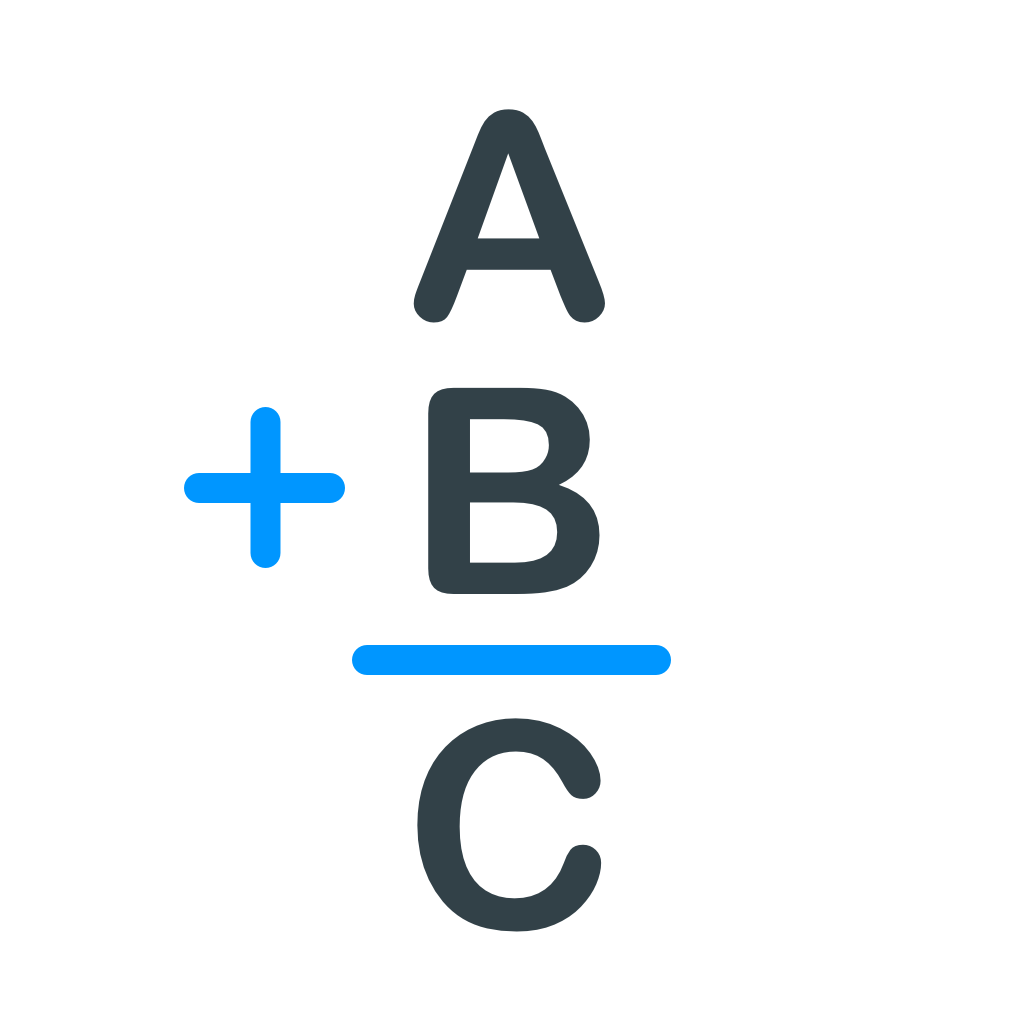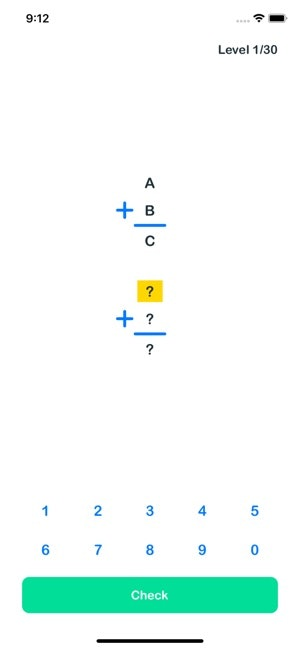# ABC Math Puzzle

Only 30 Levels. Can you solve them all?
0 reviews
Do you use ABC Math Puzzle?
What is ABC Math Puzzle?
A + B = C can be 1 + 2 = 3. A + B = C can't be 1 + 1 = 2. The same letters must be the same numbers. Different letters must be different numbers.

### Recent launches

ABC Math Puzzle
A + B = C can be 1 + 2 = 3.
A + B = C can't be 1 + 1 = 2.
The same letters must be the same numbers.
Different letters must be different numbers.